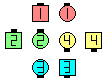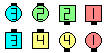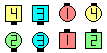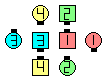Definitions of Square Dance Calls and Concepts
Interlocked Plenty [C3A]Index -->  Plus  |  A1  |  A2  |  C1  |  C2  |  C3A  |  C3B  |  C4  |  NOL  |
Definitions (Text Only) -->  Plus  |  A1  |  A2  |  C1  |  C2  |  C3A  |  C3B  |  C4  |  NOL  |
 Find call:

 \$B8@8l(B\$B0lHL2=\$5\$l\$?(B 1/4 Line \$B\$^\$?\$OB>\$NE,@Z\$J(B formation \$B\$+\$i!%(B

1. Interlocked Little (Outsides 1/4 Right and Counter Rotate 1/4 as Centers Step And Cross Fold);
2. Interlocked Little \$B\$r\$7\$F(B, Split Circulate twice (\$BIaDL(B Tandem Partner Trade \$B\$N\$h\$&\$K\$J\$j\$^\$9(B) \$B\$^\$?\$O(B Reverse The Pass \$B\$r\$7(B,
3. Outsides \$B\$O(B Counter Rotate 1/4 and Roll \$B\$r(B, Centers \$B\$O(B Concentric 1/2 Zoom \$B\$r\$7\$^\$9!%(B

\$BIaDL(B 1/4 Tag \$B\$G=*\$o\$j\$^\$9!%(B

This is a 3-part call.Interlocked Plenty\$B\$NA0(B Interlocked Little\$B\$N8e(B Split Circulate twice \$B\$N8e(B Outsides Counter Rotate 1/4 & Roll as Centers Concentric 1/2 Zoom\$B\$N8e(B (\$B=*\$o\$j(B)

\$BCm

• \$B\$b\$7(B, Split Circulates \$B\$r\$7\$?8e\$G(B, Centers \$BA40w\$,30\$r8~\$\$\$F\$\$\$?>l9g(B, U-Turn Back (set \$B\$NCf?4\$+\$iN%\$l\$k\$h\$&\$K(B) \$B\$r\$7\$F(B Touch \$B\$r\$7\$^\$9!%\$b\$7(B, Centers \$B\$,A40w8~\$+\$\$9g\$C\$F\$\$\$?\$i(B Touch \$B\$7\$^\$9!%(B
• \$BNr;KE*\$K(B, \$B%3!<%i!<\$O(B Interlocked Plenty \$B\$r(B Twin Diamonds \$B\$+\$i\$b%3!<%k\$7\$F\$*\$j(B, \$B\$3\$N>l9g(B Outsides \$B\$O(B 1/4 Right \$B\$r>JN,\$7\$^\$9!%\$3\$N;H\$\$J}\$O(B, \$B:#\$O(B '\$B\$(!
• \$BIaDL\$N(B Plenty [C1] \$B\$HF1\$8\$h\$&\$K(B, \$B%3!<%i!<\$O\$7\$P\$7\$P(B Replace the Split Circulates (\$BNr;KE*\$K\$O(B turning the Stars) \$B\$GB>\$N%3!<%k\$KCV\$-49\$(\$?\$j(B, \$B\$\$\$m\$\$\$m\$J>l=j\$G\$N(B Interrupt the Split Circulates \$B\$GB>\$N%3!<%k\$rESCf\$K3d\$j9~\$^\$;\$?\$j\$7\$^\$9!%(B(\$BLul=j\$G\$N\$H\$O(B, Interrupt Before Split Circulates \$B\$N\$h\$&\$K(B, 2 \$B\$D\$N(B Circulates \$B\$N(B "\$BA0(B/\$BCf(B/\$B8e(B" \$B\$r;X<(\$9\$k\$3\$H\$G\$9(B.)
• Interlocked Scoot & Plenty [C3A]: \$B\$G\$O(B, Outsides \$B\$O;X<(\$,\$J\$1\$l\$P(B, \$B;H\$C\$?Plenty [C1] (Lee Kopman 1972): \$B0lHL2=\$5\$l\$?(B 1/4 Tag \$B\$^\$?\$OB>\$NE,@Z\$J(B formation \$B\$+\$i!%(B Little (Outsides \$B\$O(B 1/4 Right & Counter Rotate 1/4, Centers \$B\$O(B Step & Fold) \$B\$r\$7\$F(B, Split Circulate twice \$B\$r\$7(B, Outsides \$B\$O(B Counter Rotate 1/4 and Roll, Centers \$B\$O(B Concentric 1/2 Zoom \$B\$r\$7\$^\$9!%(B \$BIaDL(B 1/4 Tag \$B\$G=*\$o\$j\$^\$9!%(B

\$BInterlocked Little [C3A] \$B\$H(B Reverse The Pass [C3B].CALLERLAB definition for Interlocked PlentyChoreography for Interlocked PlentyComments? Questions? Suggestions?